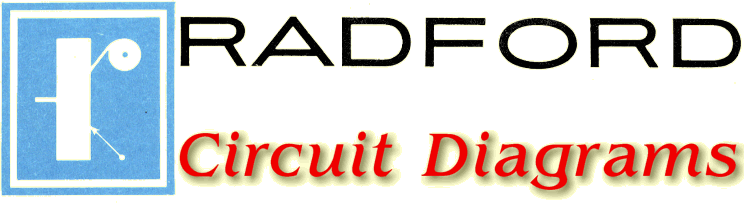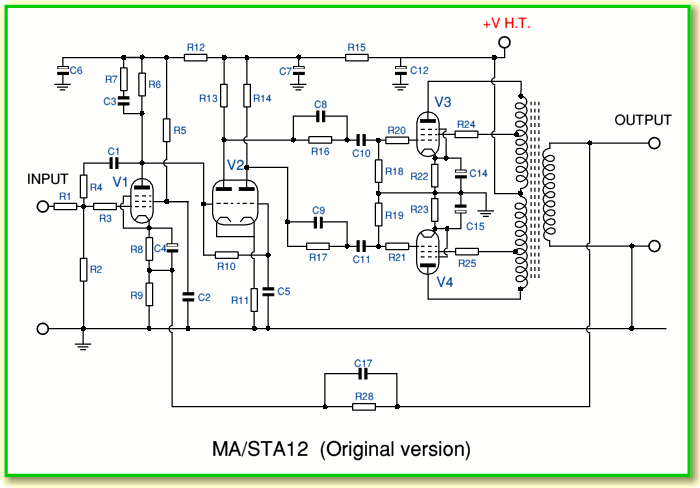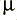Radford Electronics remain well regarded for their valve power amplifiers. This is because the amplifiers gained a well-justified reputation for outstanding performance. This webpage provides examples of the circuit diagrams of their STA (STereo Amplifier) and MA (Mono Amplifier) power amps, and outlines the development in the designs. I’ve omitted all power or voltage ratings as the circuits are not displayed for constructional purposes, but to enable study of the circuit topologies used. I’ve also omitted details of the transformers which would be vital to the actual performance. The details of the physical wiring arrangements and the ground paths would also affect performance.

The original MA/STA amplifiers appeared at the start of the 1960s The STA12 was reviewed in the March 1961 issue of ‘Hi Fi News’ magazine.The above shows a circuit diagram of the MA/STA12 (the stereo amp simply used two of the mono amplifiers with an uprated power supply.) The version shown is based on the circuit described in Hood’s Valve and Transistor Amplifiers. N.B. The diagram in Hood has some obvious errors which I have corrected. However they may be more subtle errors which I have missed in the above, and also in the values below!

Component List for original MA/STA amplifiers

 R1 = 47k R2 = 220k R3 = 4k7 R4 = 1M2 R5 = 390k R6 = 100k R7 = 4k7 R8 = 2k2 R9 = 100 R10 = 1M R11 = 82k R12 = 270k R13 = 180k R14 = 185k R15 = 15k R16 = 1M R17 = 1M R18 = 470k R19 = 470k R20 =4k7 R21 = 2k2 R22 = 470 R23 = 470 R24 = 1k R25 = 1k R28 = 2k7 C1 = 10n C2 = 0.05C3 = 1n C4 = 50C5 = 0.25C6 = 8C7 = 8C8 = 10n C9 = 10n C10 = 0.1C11 = 0.1C12 = 20C14 = 50C15 = 50C17 = 1n V1 = EF86 V2 = ECC83 V3 = EL34 V4 = EL34

In practice, as pointed out by George Tillett in the HFN review, the design was a tweaked version of the widely used Mullard 5/20. For the purpose of comparison the diagram of the 5/20 is shown below.Component List for Mullard 5/20

 R1 = 1M R2 = 4k7 R3 = 4k7 R4 = 2k2 R5 = 100 R6 = 100k R7 = 390k R8 = 82k R9 = 270k R10 = 1M R11 = 180k R12 = 180k R13 = * R14 = 470k R15 = 470k R17 = 15k R18 = 2k2 R19 = 2k2 R20 = 470 R21 = 470 R24 = 1k R25 = 1k C1 = 47p C2 = 0.05C3 = 50C4 = 8C5 = 8C6 = 0.25C9 = * C10 = 0.5C11 = 0.5C12 = 8C13 = 50C14 = 50The values marked as * should be chosen according to the load.
• 4 Ohm load R13 = 3k9, C9 = 470p
• 8 Ohm load R13 = 5k6, C9 = 330p
• 16 Ohm load R13 = 8k2, C9 = 220p

The improvements made by Arthur Radford were mainly based on his ability to produce superb output transformers. However if you compare the above designs you can see some ‘tweaks’ which were applied to the 5/20 circuit to help the MA/STA deliver improved performance. The most significant of these are in two places.
• The components labelled as C1 and R4 in the original MA/STA design apply some local anode-grid feedback to the first gain device, V1. Although this reduces the open loop gain it tends to flatten the frequency and phase response. It also reduces the output impedance of the first stage which means the second stage is less affected by its own Miller capacitance.
• The components C8, R16, C9, R17 act as gain/phase correction networks in between V2 and the output valves.

Given the good output transformers, the above changes meant that global feedback could improve the performance without risk of instability. Thus the design could achieve outstanding performance.

Although the original MA/STA design was well received, Arthur Radford felt he could do better. He therefore worked with Arthur Bailey and within a year they developed the ‘Mk2’ version.Component List for MA15 Mk 2

 R1 = 100k R2 = 100 R3 = 2k2 R4 = 330k R5 = 100k R6 = 2k7 R7 = 220k R8 = 1M R9 = 33k R10 = 8k2 R11 = 33k R12 = 39k R13 = 1M R14 = 1M R15 = 470k R16 = 470k R17 = 2k2 R18 = 2k2 R19 = 390 R20 = 390 R21 = 1k R22 = 1k R23 = 560 R24 = 3k9 R25 = 390 R26 = 2k7 R27 = 270 R28 = 1k8 R29 = 8k2 R32 = 68k C1 = 8C2 = 0.1C3 = 500p C4 = 50C5 = 2C6 = 1C7 = 4n7 C8 = 0.1C9 = 0.1C10 = 16C11 = 50C12 = 50C13 = 20C15 = 270p C16 = 1n C17 = 340p V1 = EF86 V2 = 6U8* V3 = EL34 V4 = EL34

* V2 may also be an ECF82

The above lists the values as given for the Hi Fi News constructional version of the MA15. This appeared in a series of article from June to September 1962. Note that R32 was an addition made in the last article, so isn’t shown in the diagram in the June 1962 issue. To change the design into an MA25 Mk2 the following changes were made.

Component List changes specified in HFN for MA25 Mk2

 R19 = 470 R20 = 470 C3 = 680p C13 = 40V3 = KT88 V4 = KT88

The changes that distinguish the Mk2 from the original versions are in two areas
• A better output transformer.
• The phase splitter stage (V2) uses a pentode-triode instead of a double-triode.

The change to a pentode-triode was a development by Arthur Bailey and has a dramatic effect on the open loop gain of the amplifier. In effect, although the double-triode only had a flat gain bandwidth of around 15 kHz, the pentode-triode extended this to above 150kHz. As with the previous design, this flattening of the open loop response in terms of amplitude and phase made the amplifier inherently better, and allowed global feedback to provide further improvements without the amplifier oscillating on some loudspeaker loads.